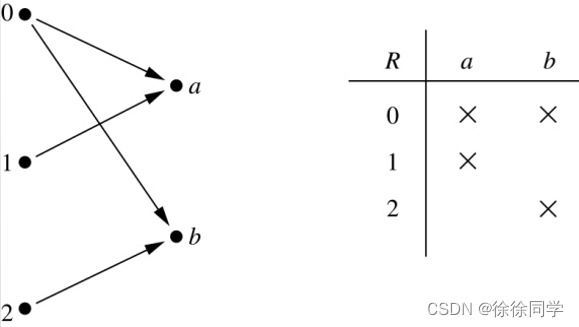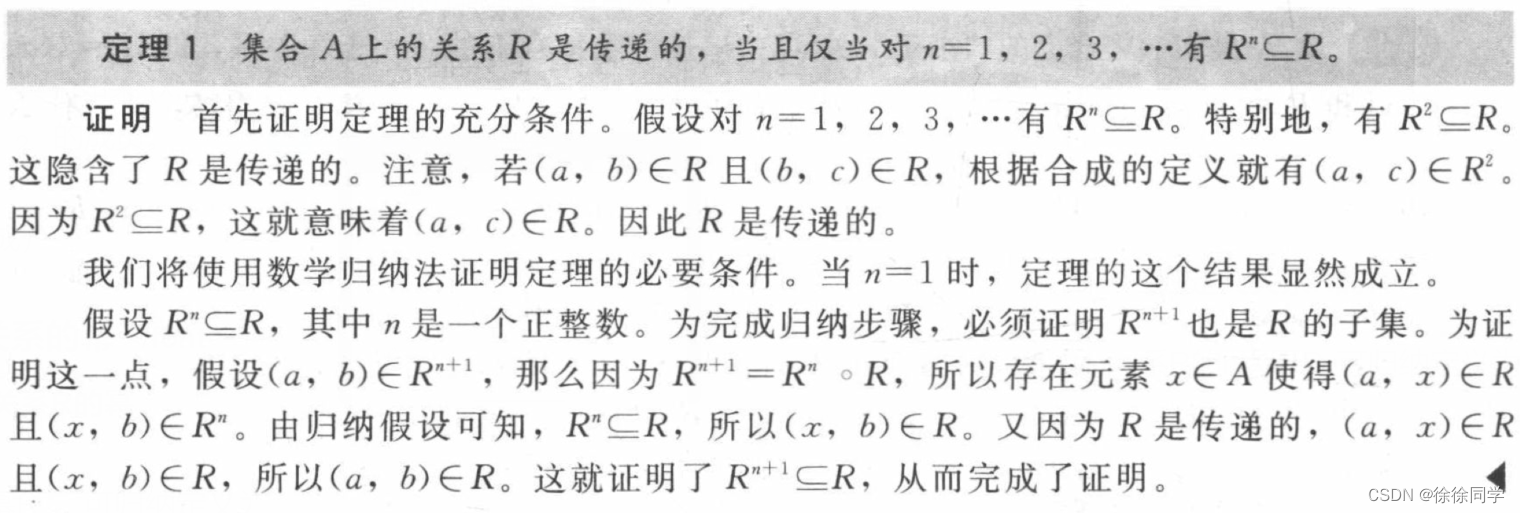## 离散数学_九章：关系（1）

article2023/9/26 21:30:09/

### 关系

• 9.1关系及其性质
• 1、二元关系
• 2、集合A上的关系
• 3、n元素集合 有多少个关系？
• 4、关系的性质
• 1. 自反
• 2. 对称
• 3. 反对称
• 4. 传递
• 5、关系的组合
• 关系的合成
• 关系的幂

# 9.1关系及其性质

## 1、二元关系

🐳换句话说，一个从A到B的二元关系是集合R，其中每个有序对的第一个元素取自A而第二个元素取自B。

📘例：设 A = { 0, 1, 2 }， B = { a, b }

{ (0, a), (0, b), (1, a), (2, b) }是一个从 A 到 B 的关系。## 2、集合A上的关系

🐳换句话说，集合A上的关系是从A到A的关系

📘例：设 A = { a, b, c }

## 3、n元素集合 有多少个关系？

A上的关系和 A × A 的子集是一回事
➡所以可以直接计数 A × A 的子集。

📘例：设 B = { b }

📘例：设 C = { a, b, c }

## 4、关系的性质

### 1. 自反

（这里的论域是A中所有元素的集合。）

📘例：

R1 = { (a, b) | a ≤ b }，
R3 = { (a, b) | a = b 或 a = – b }，
R4 = { (a, b) | a = b }。

R2 = { (a, b) | a > b }（注意3 ≯ 3），
R5 = { (a, b) | a = b + 1 }（注意3 ≠ 3 + 1），
R6 = { (a, b) | a + b ≤ 3 }（注意4 + 4 ≰ 3）。

### 2. 对称

📘例：

R3 = { (a, b) | a = b 或 a = – b }，
R4 = { (a, b) | a = b }，
R6 = { (a, b) | a + b ≤ 3 }。

R1 = { (a, b) | a ≤ b }（反例：3 ≤ 4，但4 ≰ 3），
R2 = { (a, b) | a > b }（反例：4 > 3，但 3 ≯ 4），
R5 = { (a, b) | a = b + 1 }（反例：4 = 3 + 1，但3 ≠ 4 + 1）。

### 3. 反对称

📘例：

R1 = { (a, b) | a ≤ b }，
R2 = { (a, b) | a > b }，
R4 = { (a, b) | a = b }，
R5 = { (a, b) | a = b + 1 }。

R3 = { (a, b) | a = b 或 a = – b }（反例： (1, – 1) 和 (–1, 1) 都属于R3），
R6 = { (a, b) | a + b ≤ 3 }（反例： (1, 2) 和 (2, 1) 都属于R6）。

💻对称与反对称的概念不是对立的，因为一个关系可以同时有这两种性质或者两种性质都没有。

### 4. 传递

📘例：

R1 = { (a, b) | a ≤ b }，
R2 = { (a, b) | a > b }，
R3 = { (a, b) | a = b 或 a = – b }，
R4 = { (a, b) | a = b }。

R5 = { (a, b) | a = b + 1 }
（反例：(3, 2) 和 (4, 3) 都属于R5，但不属于 (3, 3)），
R6 = { (a, b) | a + b ≤ 3 }
（反例：(2, 1) 和 (1, 2) 都属于R6，但不属于 (2, 2)）。

## 5、关系的组合

### 关系的合成

R2 和 R1 的合成是 A 到 C 的关系，其中如果 (x, y) 是 R1 的成员，(y, z) 是 R2的成员，那么 (x, z) 则是 R1 ∘ R2 的成员。

（R1 ∘ R2 表示R1 和 R2 的合成）

### 关系的幂### CentOS7-部署Tomcat并运行Jpress

1. 简述静态网页和动态网页的区别。 2. 简述 Webl.0 和 Web2.0 的区别。 3. 安装tomcat8&#xff0c;配置服务启动脚本&#xff0c;部署jpress应用。1、简述静态网页和动态网页的区别 静态网页&#xff1a; 请求响应信息&#xff0c;发给客户端进行处理&#xff0c;由浏览器进…

### 4个 Python 库来美化你的 Matplotlib 图表

Matplotlib是一个被广泛使用的Python数据可视化库&#xff0c;相信很多人都使用过。 但是有时候总会觉得&#xff0c;Matplotlib做出来的图表不是很好看、不美观。 今天我就给大家分享四个美化Matplotlib图表的Python库&#xff0c;它们可以轻松让你的Matplotlib图表变得好看…

### ( “树” 之 DFS) 404. 左叶子之和 ——【Leetcode每日一题】

404. 左叶子之和 给定二叉树的根节点 root &#xff0c;返回所有左叶子之和。 示例 1&#xff1a; 输入: root [3,9,20,null,null,15,7] 输出: 24 解释: 在这个二叉树中&#xff0c;有两个左叶子&#xff0c;分别是 9 和 15&#xff0c;所以返回 24 示例 2: 输入: root …

### ESP32设备驱动-SHT20温湿度传感器驱动

SHT20温湿度传感器驱动 文章目录 SHT20温湿度传感器驱动1、SHT20介绍2、硬件准备3、软件准备4、驱动实现1、SHT20介绍 Sensirion 的 SHT20 湿度和温度传感器已成为外形尺寸和智能方面的行业标准：嵌入在 3 x 3mm 封装和 1.1mm 高度的可回流焊双扁平无引线 (DFN) 封装中，它提供…